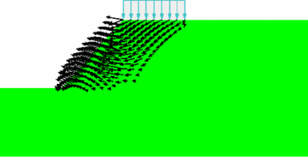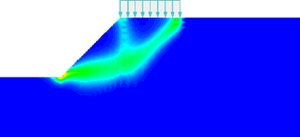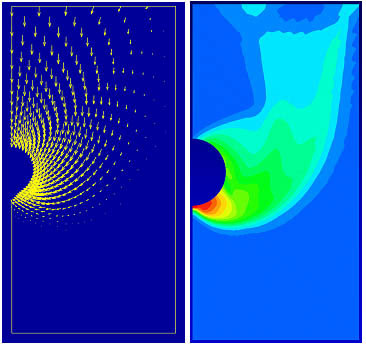Priority Research Centre for
GEOTECHNICAL SCIENCE AND ENGINEERING# Computational Limit Analysis of Geostructures

## Aims and Background

Geotechnical stability analysis is traditionally performed by a variety of approximate methods that are based on the theory of limit equilibrium. Although they are simple and appeal to engineering intuition, these techniques suffer from a number of serious disadvantages, not the least of which is the need to presuppose an appropriate failure mechanism in advance. This feature can lead to inaccurate predictions of the true failure load, especially for realistic problems involving layered materials, complex loading, or three-dimensional deformation.

A much more rigorous method for assessing the stability of geostructures became available with the advent of the limit (or bound) theorems of classical plasticity in the 1950s. These theorems can be used to give upper and lower bounds on the predicted collapse load (a most valuable property in practice), do not require assumptions to be made about the mode of failure, and use only simple strength parameters that are familiar to geotechnical engineers. Although many ingenious bound results have been derived using analytical or numerical methods, practical application of the limit theorems has been restricted by the need to develop specific solution strategies for each problem. Over the last decade, the Newcastle Geotechnical Research Group has developed powerful new methods for performing stability analysis that combine the limit theorems with finite elements and optimisation. These methods are very general and can deal with layered soil profiles, anisotropic strength characteristics, complicated boundary conditions, and complex loading in both two and three dimensions. Indeed, they have already been used to obtain new stability solutions for a wide range of practical problems including soil anchors, slopes, foundations under combined loading, excavations, tunnels, mine workings, and sinkholes.Stability of Footing Above Slope - Failure MechanismStability of Footing Above Slope - Equivalent Plastic Strain

## Finite element formulations of the upper and lower bound theorems

Computational limit analysis is based on the upper and lower bounds theorems of classical plasticity. Each of these theorems involves certain constraints which, if fulfilled, lead to rigorous bounds on the collapse load. The requirements of the upper and lower bound theorems (static/kinematic admissibility) call for specialised finite element formulations. Such formulations have been developed for general 3D solids as well as for beams and laterally loaded plates. A notable feature in these methods is the ability to include discontinuities in the stress field for lower bound analysis and discontinuities in the velocity field for upper bound analysis.

Although the upper and lower bound theorems appear to be quite different in their original form, involving for example two different sets of physical quantities (stresses and velocities respectively), they are in fact duals of each other. This fact can be exploited in numerical formulations to unify the two types of analyses. The result is a general format which allows for upper and lower bound limit analysis of materials with arbitrary convex yield criteria.

## Optimization algorithms

The upper and lower bound theorems lead naturally to nonlinear convex optimization problems which, depending on the mesh density, may be very large. A key issue in computational limit analysis is the solution of these problems efficiently and robustly. Over the years a number of different strategies have been employed. The earliest algorithms considered were based on the well known simplex algorithm of linear programming, but these formulations had a major limitation in that the yield surface had to be linearized. More recently, various interior-point methods have been developed and adapted specifically to the types of problems which arise in the limit analysis of geomaterials. These methods do not require linearization of the yield surface and are in principle applicable to any convex yield criterion. More recently, so-called conic programming methods have been developed. These are applicable to a narrower range of yield criteria but have a superior performance in terms of efficiency and robustness. The yield criteria that can be considered using conic programming include the von Mises, Drucker-Prager and Mohr-Coulomb criteria.

Although the finite element formulations developed are rather efficient and capable of producing tight bounds with a moderate computational effort, the procedures have been enhanced by adaptive remeshing procedures. The problem under consideration is first solved on a coarse mesh. An estimate of the error is then computed and on the basis of this error a new mesh is constructed. This procedure is typically repeated four or five times and has shown to be very efficient. The key issue is the formulation of reliable error estimators and a number of non-conventional strategies have been developed which perform very well in practice. An example of a typical limit analysis computation using adaptive remeshing is shown in the figure below.

## ApplicationsAbove: Footing - last three steps in an adaptive upper bound limit analysis procedure and power dissipation (far right)

The methods developed have been applied successfully to a large number of problems in geotechnics. These include slopes, excavations, anchors, retaining walls, and tunnels – in 2D as well as in 3D.Above: Tunnel in a purely cohesive material – collapse mechanism (left) and power dissipation (right)Above: Comparison of upper and lower bound tunnel stability numbers with experimental resultsAbove: Typical finite element mesh (coarse) for three-dimensional stability of plate anchor in clay